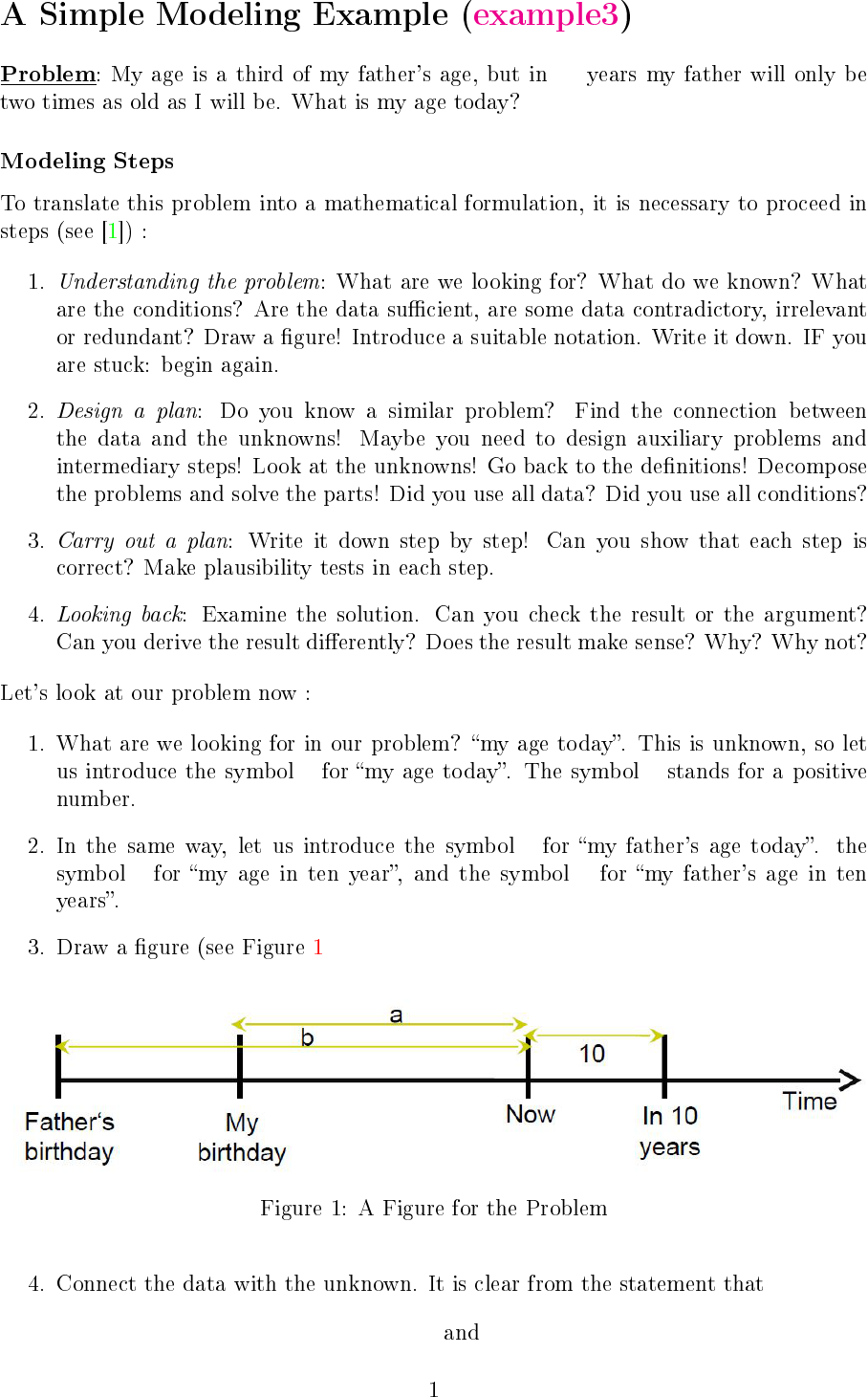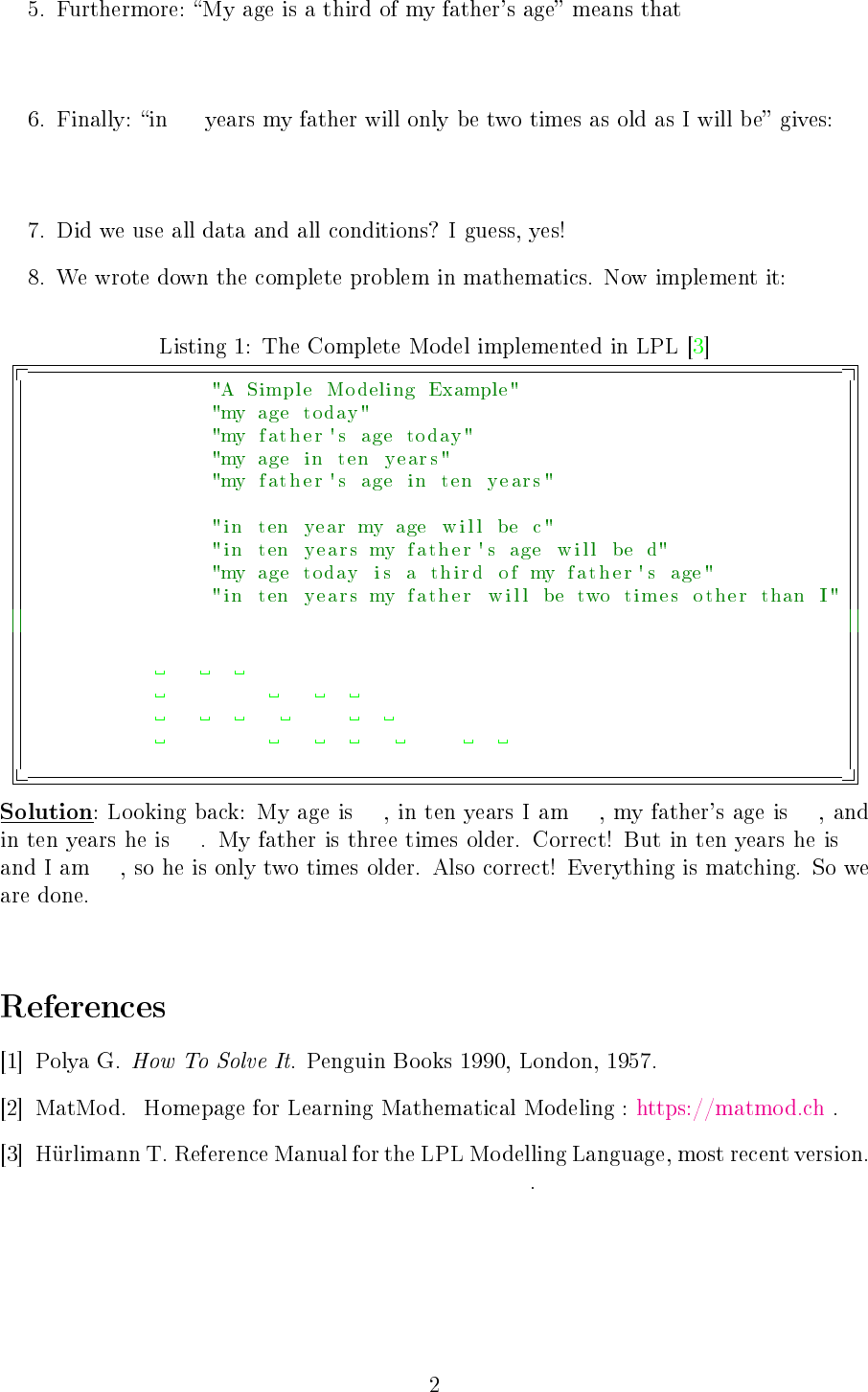10
a a
b
c d
c = a + 10 d = b + 10a = b/3
10
d = 2c
model exercise3 ;
variable a ;
b ;
c ;
d ;
constraint
A: c = a+10 ;
B: d = b+10 ;
C: a = b/3 ;
D: d = 2
*
c
;
solve;
Write('My age is %d\n', a);
Write('My father\'s age is %d\n', b);
Write('My age in ten years is %d\n', c);
Write('My father\'s age in ten years is %d\n', d);
end
10 20 30
40 40
20
https://matmod.ch/lpl/doc/manual.pdf